# IBPS PO MAINS – 2018 : APTITUDE QUESTIONS

Dear Bankersdaily Aspirants,

Hope all are getting ready for IBPS PO MAINS examination.As long as we Practice the Question sets we will be getting accuracy in the examination.Hence we are providing you some of the important practice question sets for your practice which will make you score good in all the upcoming examinations including IBPS PO MAINS.

Here is some of the aptitude questions for your practice.All the best for all the aspirants whoever is appearing for the IBPS PO MAINS Examination.

QUANTITATIVE APTITUDE

D.1-5) Find the missing number in the following series.

Q.1) 16, 40, 140, 630, 3465, ?

a) 25222.5

b) 22525.5

c) 22522.5

d) 22252.5

e) None of these

Q.2) 405, 270, 180, 120, ?

a) 40

b) 60

c) 80

d) 70

e) 90

Q.3) 15, 16, 20, 9, 25, 2, ?

a) 41

b) 49

c) 30

d) 31

e) 35

Q.4) 7, 15, 115, 142, 223, 287, ?

a) 351

b) 341

c) 799

d) 499

e) None of these

Q.5) 45, 51, 60, 75, 99, ?

a) 125

b) 140

c) 130

d) 135

e) 120

D.6-10) Study the following information carefully and answer the following questions.

The following table shows the production of wheat (in million tonnes) across 5 states over the given years.

 States/years 2000 2001 2002 2003 2004 Punjab 540 550 530 525 510 Haryana 520 490 510 490 500 Madhya Pradesh 450 420 480 510 490 Maharashtra 380 400 440 460 450 Bihar 500 520 490 510 480

Q.6) What is the average production of wheat in the year 2001 for all the states together (million tonnes)?

a) 474

b) 476

c) 482

d) 496

e) None of these

Q.7) What is the ratio of the production of wheat in Maharashtra in the years 2002 and 2003 together to the production of wheat in Madhya Pradesh for the same years?

a) 12:11

b) 11:10

c) 10:11

d) 9:10

e) 10:9

Q.8) Production of wheat in Bihar in the years 2003 and 2004 together is approximately what percentage of the production of wheat in Punjab in the same years?

a) 94%

b) 89%

c) 90%

d) 96%

e) None of these

Q.9) What is the total production of wheat in Punjab over the given period?

a) 2655

b) 2565

c) 2665

d) 2566

e) None of these

Q.10) What is the difference between the total production of wheat in the year 2003 and the total production of wheat in the year 2004 (in million tonnes)?

a) 75

b) 65

c) 60

d) 55

e) 70

D.11-15) What is the approximate value will come in place of ‘?’ in the following questions?

Q.11) 68% of 249.09-44.99×5.92+129.98% of 501=?-150

a) 600

b) 400

c) 700

d) 500

e) 800

Q.12) (8.91)3-(7.03)3-(11.92)2+(15.04)2=?

a) 311

b) 467

c) 1235

d) 476

e) 367

Q.13)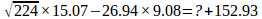a) 171

b) -121

c) 121

d) -171

e) -21

Q.14) 55.08×19.91-40.06×25.08=? ×9.95

a) 50

b) 100

c) 10

d) 9

e) 12

Q.15)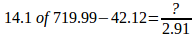a) 70

b) 90

c) 80

d) 75

e) 65

D.16-20) Study the following information carefully and answer the given questions.

The following bar graph shows cost price (CP) and selling price (SP) of 5 different items (in Rs.).Profit=SP-CP

Loss=CP-SP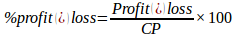Q.16) In which item profit percentage is maximum?

a) Item 1

b) Item 3

c) Item 5

d) Both Items 1 and 4

e) Item 4

Q.17) What is the ratio between the selling price of items 1 and 2 together to cost price of the same items?

a) 13:11

b) 97:95

c) 96:95

d) 12:13

e) None of these

Q.18) Profit percentage of item 3 is what percentage of the profit percentage of item 4?

a) 40%

b) 30%

c) 25%

d) 35%

e) 45%

Q.19) If the selling price of item 5 is increased by 20% from its previous selling price, then find the profit or loss percentage?

a) 20%

b) 15%

c) 25%

d) No profit or Loss

e) None of these

Q.20) What is the difference between the total profit and total loss for all the given items?

a) Rs.50

b) Rs.40

c) Rs.30

d) Rs.60

e) None of these

## Explanations

Q.1) c

×2.5 ×3.5 ×4.5 ×5.5 ×6.5

Q.2) c

× 2/3 ×2/3 ×2/3 ×2/3 ×2/3

Q.3) c

There are two series 15, 20, 25 (difference 5) so next is 30

Another series is 16, 9, 2 (subtract 7)

Q.4) a

+23 +102 +33 +92 +43 +82

Q.5) d

+6 +9 +15 +24 +36

+3 +6 +9 +12

Q.6) b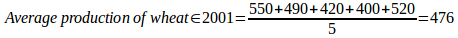Q.7) c

Required ratio= (440+460): (480+510) =900: 990=10:11

Q.8) d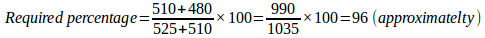Q.29) a

Total production in Punjab=540+550+530+525+510=2655 million tonnes

Q.10) b

Required difference

= (525+490+510+460+510)-(510+500+490+450+480) =2495-2430

=65 million tonnes

Q.11) c

68% of 249.09-44.99×5.92+129.98% of 501=?-150

?=170-270+650+150=700

Q.12) b

(8.91)3-(7.03)3-(11.92)2+ (15.04)2=?

?= 729-343-144+225=467

Q.13) d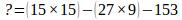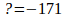Q.14) c

55.08×19.91-40.06×25.08=? ×9.95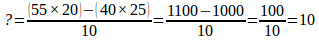Q.15) b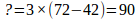Q.16) a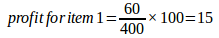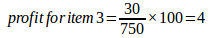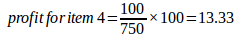Q.17) c

Required ratio= (460+500): (400+550) = 960:950= 96:95

Q.18) b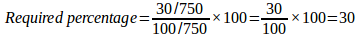Q.19) d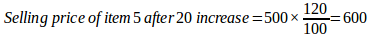Cost price is equals to the selling price

Therefore No profit or loss

Q.20) b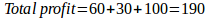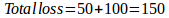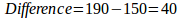## Other Important Notification: Released In October 2018

### IBPS SO 2018 Notification Released: 1599 Vacancies